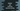# Python example to print the function name as string with __name__ and __qualname__## How to get the function name as string:

In this post, we will learn how to get the name of a function as string in Python. Python provides an easy way to get the function name. Python provides two attributes called `__name__` and `__qualname__` to get the name of a function in string format. Let’s learn how to read these attributes with examples.

### How to use the `__name__` attribute to read a function name in Python:

The `__name__` attribute is available for each function in Python. We can use the function name and `.__name__` to get the name of that function. Let’s try this with an example:

``````def first_function():
print('Hello from first_function')

def second_function():
print('Hello from second_function')

print(first_function.__name__)
print(second_function.__name__)``````

If you run this program, it will print the names of the functions first_function and second_function:

``````\$ python3 example.py
first_function
second_function``````

### Type of `__name__`:

Let’s check the type of the valur returned by `__name__`. We can use the type() method to get that:

``````def first_function():
print('Hello from first_function')

print(type(first_function.__name__))``````

It will print the type of `first_function.__name__`:

``<class 'str'>``

### `__name__` with system modules:

We can use `__name__` with system modules or any other modules as well. It will return the name of that module:

``````import os
import random

print(os.__name__)
print(random.__name__)``````

It will print:

``````os
random``````

### How to read the `__qualname__` attribute to read a function name:

Python provides one more attribute called `__qualname__` to read a function name. It is also called as qualified name. Note that it is available since Python 3.3.

``````def first_function():
print('Hello from first_function')

print(first_function.__qualname__)``````

It will print:

``first_function``

`__qualname__` is better than `__name__` if you use it for debugging purpose. Let’s take a look at the below example:

``````class Parent:
def print_hello():
print('Hello')
class Child:
def print_hello():
print('Hello')

print(Parent.print_hello.__name__)
print(Parent.Child.print_hello.__name__)``````

This is printing the name of the print_hello methods of the Parent and Child classes. But since the name is not different of these methods, it will print the same for both:

``````print_hello
print_hello``````

Now, let’s try it with `__qualname__`:

``````class Parent:
def print_hello():
print('Hello')
class Child:
def print_hello():
print('Hello')

print(Parent.print_hello.__qualname__)
print(Parent.Child.print_hello.__qualname__)``````

It will print the full path with the name:

``````Parent.print_hello
Parent.Child.print_hello``````

So, if you want to use these attributes for logging, `__qualname__` will give a better result.